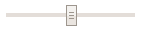OpenCV  4.1.0 Open Source Computer Vision

## Goal

• Use HTML DOM Input Range Object to add a trackbar to your application.

## Code Demo

Here, we will create a simple application that blends two images. We will let the user enter the weight by using the trackbar.

First, we need to create three canvas elements: two for input and one for output. Please refer to the tutorial Getting Started with Images.

Then, we use HTML DOM Input Range Object to implement the trackbar, which is shown as below.Note
<input> elements with type="range" are not supported in Internet Explorer 9 and earlier versions.

You can create an <input> element with type="range" with the document.createElement() method:

let x = document.createElement('INPUT');
x.setAttribute('type', 'range');

You can access an <input> element with type="range" with getElementById():

let x = document.getElementById('myRange');

As a trackbar, the range element need a trackbar name, the default value, minimum value, maximum value, step and the callback function which is executed everytime trackbar value changes. The callback function always has a default argument, which is the trackbar position. Additionally, a text element to display the trackbar value is fine. In our case, we can create the trackbar as below:

Weight: <input type="range" id="trackbar" value="50" min="0" max="100" step="1" oninput="callback()">
<input type="text" id="weightValue" size="3" value="50"/>

Finally, we can use the trackbar value in the callback function, blend the two images, and display the result.

let weightValue = document.getElementById('weightValue');
let trackbar = document.getElementById('trackbar');
weightValue.setAttribute('value', trackbar.value);
let alpha = trackbar.value/trackbar.max;
let beta = ( 1.0 - alpha );